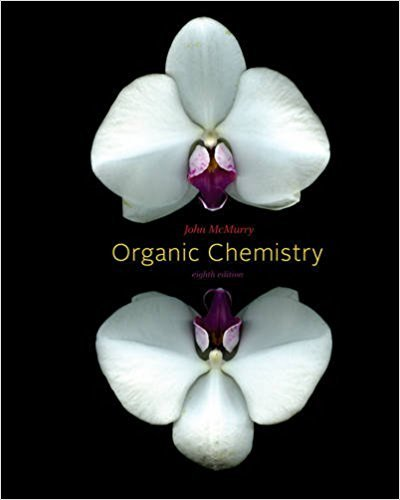×
×

# Tell how many hydrogens are bonded to each carbon in the following compounds, and giveISBN: 9780840054449 463

## Solution for problem 1.15 Chapter 1.12

Organic Chemistry | 8th Edition

• Textbook Solutions
• 2901 Step-by-step solutions solved by professors and subject experts
• Get 24/7 help from StudySoup virtual teaching assistantsOrganic Chemistry | 8th Edition

4 5 1 418 Reviews
18
3
Problem 1.15

Tell how many hydrogens are bonded to each carbon in the following compounds, and give the molecular formula of each substance: Adrenaline Estrone (a hormone) (a) NHCH3 HO HO OH (b

Step-by-Step Solution:
Step 1 of 3

Chapter 13 : Simple linear regression Book : Introductory Statistics , Prem S.Mann 8e Explaining Important More Important Learning objectives : 13.1 Simple Linear Regression 13.2 Standard deviation of errors and coefficient of Determination 13.3 Inferences about B 13.4 Linear Correlation 1) Simple Linear Regression : 13.1.1 Simple Regression : is a mathematical equation that...

Step 2 of 3

Step 3 of 3

##### ISBN: 9780840054449

Unlock Textbook Solution